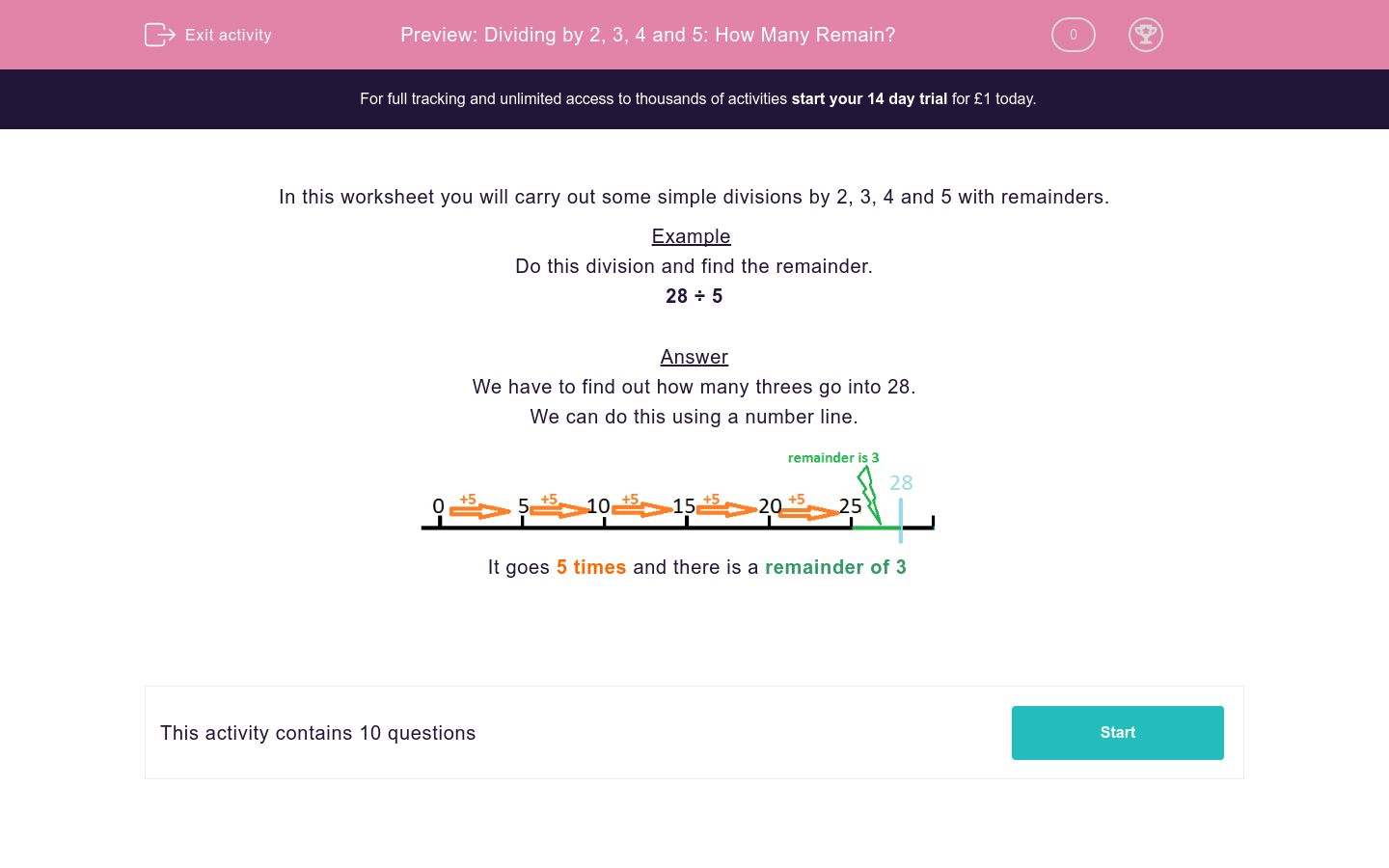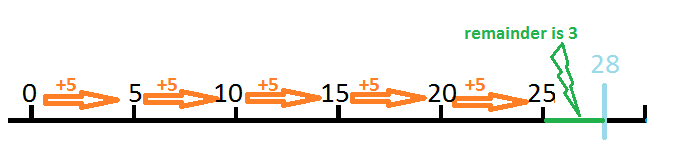# Dividing by 2, 3, 4 and 5: How Many Remain?

In this worksheet, students divide two-digit numbers by 2, 3, 4 and 5. They note down any remainders.Key stage:  KS 2

Curriculum topic:   Maths and Numerical Reasoning

Curriculum subtopic:   Mixed Problems

Difficulty level:### QUESTION 1 of 10

In this worksheet you will carry out some simple divisions by 2, 3, 4 and 5 with remainders.

Example

Do this division and find the remainder.

28 ÷ 5

We have to find out how many threes go into 28.

We can do this using a number line.It goes 5 times and there is a remainder of 3

Work out the division below.

21  ÷  4  =
 A
remainder
 B

## Column B

B
5
A
1

Work out the division below.

41  ÷  5  =
 A
remainder
 B

## Column B

B
1
A
8

Work out the division below.

20  ÷  3  =
 A
remainder
 B

## Column B

B
2
A
6

Work out the division below.

39  ÷  4  =
 A
remainder
 B

## Column B

A
9
B
3

Work out the division below.

35  ÷  5  =
 A
remainder
 B

## Column B

B
0
A
7

Work out the division below.

34  ÷  5  =
 A
remainder
 B

## Column B

B
4
A
6

Work out the division below.

52  ÷   5   =
 A
remainder
 B

## Column B

A
10
B
2

Work out the division below.

38  ÷  3  =
 A
remainder
 B

## Column B

B
12
A
2

Work out the division below.

19 ÷  2   =
 A
remainder
 B

## Column B

B
9
A
1

Work out the division below.

49  ÷  3  =
 A
remainder
 B

## Column B

A
16
B
1
• Question 1

Work out the division below.

21  ÷  4  =
 A
remainder
 B

## Column B

B
1
A
5
• Question 2

Work out the division below.

41  ÷  5  =
 A
remainder
 B

## Column B

B
1
A
8
• Question 3

Work out the division below.

20  ÷  3  =
 A
remainder
 B

## Column B

B
2
A
6
• Question 4

Work out the division below.

39  ÷  4  =
 A
remainder
 B

## Column B

A
9
B
3
• Question 5

Work out the division below.

35  ÷  5  =
 A
remainder
 B

## Column B

B
0
A
7
• Question 6

Work out the division below.

34  ÷  5  =
 A
remainder
 B

## Column B

B
4
A
6
• Question 7

Work out the division below.

52  ÷   5   =
 A
remainder
 B

## Column B

A
10
B
2
• Question 8

Work out the division below.

38  ÷  3  =
 A
remainder
 B

## Column B

B
2
A
12
• Question 9

Work out the division below.

19 ÷  2   =
 A
remainder
 B

## Column B

B
1
A
9
• Question 10

Work out the division below.

49  ÷  3  =
 A
remainder
 B

## Column B

A
16
B
1
---- OR ----

Sign up for a £1 trial so you can track and measure your child's progress on this activity.

### What is EdPlace?

We're your National Curriculum aligned online education content provider helping each child succeed in English, maths and science from year 1 to GCSE. With an EdPlace account you’ll be able to track and measure progress, helping each child achieve their best. We build confidence and attainment by personalising each child’s learning at a level that suits them.

Get started